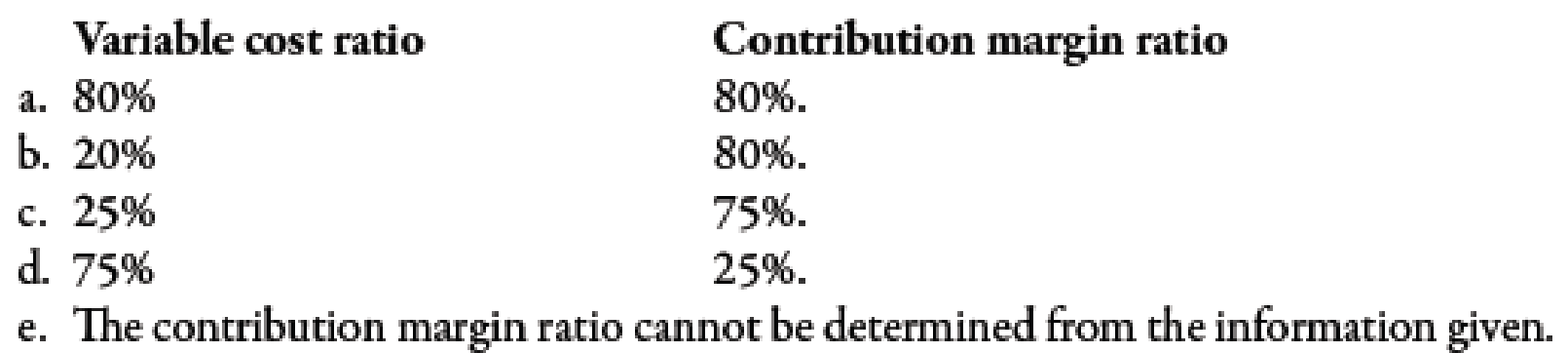Chapter 7, Problem 10MCQ### Managerial Accounting: The Corners...

7th Edition
Maryanne M. Mowen + 2 others
ISBN: 9781337115773

#### Solutions

Chapter
Section### Managerial Accounting: The Corners...

7th Edition
Maryanne M. Mowen + 2 others
ISBN: 9781337115773
Textbook Problem
33 views

# Dartmouth Company produces a single product with a price of $12, variable cost per unit of$3, and total fixed cost of $7,200.Refer to the information for Dartmouth above. The variable cost ratio and the contribution margin ratio for Dartmouth areTo determine Identify variable cost ratio and contribution margin ratio. Explanation Variable Cost Ratio: Variable cost ratio can be evaluated by dividing the total amount of variable cost by the sales. It can also be determined by dividing the variable cost per unit by the selling price per unit. Contribution Margin Ratio: The sales percentage remaining after covering the amount of total variable cost is known as the contribution margin ratio. It is the available sales dollar percentage which will be used to cover the total fixed cost. c. Use the following formula to calculate variable cost ratio: Variablecostratio=VariablecostperunitPriceperunit Substitute$3 for variable cost per unit and $12 for price per unit the above formula. Variablecostratio=$3$12=0.25 Variable cost ration is 25%. Use the following formula to calculate contribution margin ratio: Contributionmarginratio=ContributionmarginperunitPriceperunit Substitute$91 for contribution margin per unit and \$12 for price per unit the above formula

### Still sussing out bartleby?

Check out a sample textbook solution.

See a sample solution

#### The Solution to Your Study Problems

Bartleby provides explanations to thousands of textbook problems written by our experts, many with advanced degrees!

Get Started